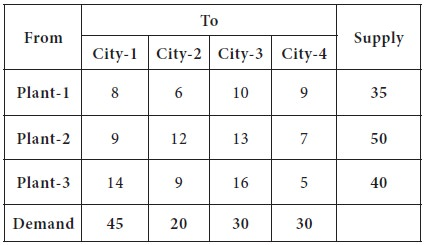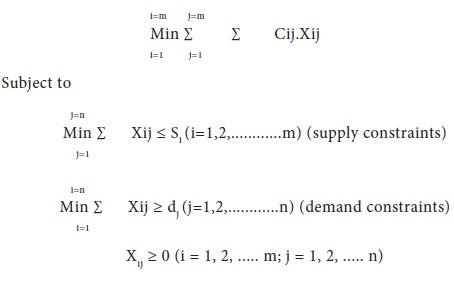Home | ARTS | Operations Management | Problem Formulation -Example - Transportation Problem

# Problem Formulation -Example - Transportation Problem

Posted On :  23.06.2018 12:35 am

Consider the following example: Adani Power Limited, which is a electric power producing company in India, has three electric power plants that supply the needs of four cities. Each power plant can supply the following numbers of kWhr of electricity: Plant 1 – 35 million; Plant 2 – 50 million; Plant 3 – 40 million.

Problem Formulation -Example

Consider the following example: Adani Power Limited, which is a electric power producing company in India, has three electric power plants that supply the needs of four cities. Each power plant can supply the following numbers of kWhr of electricity: Plant 1 – 35 million; Plant 2 – 50 million; Plant 3 – 40 million.

The peak power demands in these cities, which occur at the same time, are as follows (in kWhr): City 1 – 45 million; City 2 – 20 million; City 3 – 30 million; City 4 – 30 million. The costs of sending 1 million kWhr of electricity from plant to city depend on the distance the electricity must travel (see Table).Formulate this problem to minimize the cost of meeting each city’s peak power demand.

Solution

We begin by defining a variable for each decision that Adani Power has to make.

We define, (for i = 1, 2, 3 and j = 1, 2, 3, 4)

Xij = number of (million) kWhr produced at plant i and sent to city j

Then, the objective of the Adani Power is to minimize the total transmission cost; this is achieved by allocating appropriately by linking the plant and city through the lowest cost of transmission.

As we have discussed in the Mathematical Modelling of Problems, in the Unit-2, we derive the following objective function;

Min Z = 8X11 + 6X12 + 10X13 + 9X14 + 9X21 + 12X22 + 13X23 + 7X24 + 14X31 + 9X32 + 16X33 + 5X34

This objective can be realized subject to the following constraints.

 X11 + X12 + X13 + X14 ≤ 35 ------ (Supply constraint from Plant-1) X21 + X22 + X23 + X24 ≤ 50 ------ (Supply constraint from Plant-2) X31 + X32 + X33 + X34 ≤ 40 ------ (Supply constraint from Plant-3) X11 + X21 + X31 ≥ 45 ----- (Demand constraint at the city-1) X12 + X22 + X32 ≥ 20 ----- (Demand constraint at the city-2) X13 + X23 + X33 ≥ 30 ----- (Demand constraint at the city-3) X14 + X24 + X34 ≥ 30 ----- (Demand constraint at the city-4) And obviously, Xij ≥ 0
This is a special case of LP problem. It can be solved by the simplex algorithm, but specialized algorithms are much more efficient.

In general, a transportation problem is specified by the following parameters:

1.      A set of ‘m’ supply points from which a good is shipped. Supply point ‘i’ can supply, at most, Si units (in the above situation, m =3, S1 = 35, S2 = 50, S3 = 40)

2.      A set of ‘n’ demand points to which the good is shipped. Demand point ‘j’ must receive at least dj units of the shipped goods (you can see above, n = 4; d1 = 45, d2 = 20, d3 = 30, d4 = 30).

3.      Each unit produced at supply point ‘i’ and shipped to demand point ‘j’ incurs a variable cost cij. (In the above case, for example, c12 = 6).

Let Xij = number of units shipped from supply point i to demand point j.

Thus, a general formulation is:As we mentioned earlier, if ∑ Si = ∑ dj; that is supply equal demand, it is said to be balanced transportation problem. Adani issue is a balanced transportation problem.

Thus, for a balanced transportation problem,Solution of balanced transportation problem is simpler, therefore, it is desirable to formulate a transportation problem as a balanced transportation problem.

Thus, a transportation problem is specified by the supply, the demand, and the shipping costs, so the relevant data can be summarized in a transportation tableau. The cell in row i and column j corresponds to the variable Xij.

If Xij is a basic variable, its value is normally placed in the middle corner of the ijth cell of the tableau. The costs are normally shown in the upper right corner. The following tableau is for the Adani Power problem. (The optimal solution values are also given, which we are going to disucss shortly).Deriving the solution for a transportation problem is done in two stages; in the first stage, we try to obtain an initial basic feasible solution; in the stage-2, we try to check, whether the solution obtained in stage-1 is optimal or not; if not optimum, we modify the allocations made [known as MODI Method / u-v method].

There are many methods for finding such a starting Basic Feasible Solution [BFS]. The easier ones are the northwest-corner method, the column minima method, the row-minima method and the matrix minima method (or least cost method).

Tags : Operations Management - Transportation / Assignment & Inventory Management
Last 30 days 2500 views